R语言中的多种决策树算法实现¶

• CART，包含在 rpart 包中
• J48，包含在 RWeka 包中
• C5.0，包含在 C50 包中

Tip

可视化¶

partykit 包¶

library(partykit)
plot(as.party(rpartFit$finalModel))maptree 包¶ library(maptree) draw.tree(rpartFit$finalModel)rpart.plot 包¶

library(rpart.plot)
rpart.plot(rpartFit$finalModel, fallen.leaves = FALSE)另一个是更一般的prp()，需要自定义的参数更多，更灵活 prp(rpartFit$finalModel)混淆矩阵¶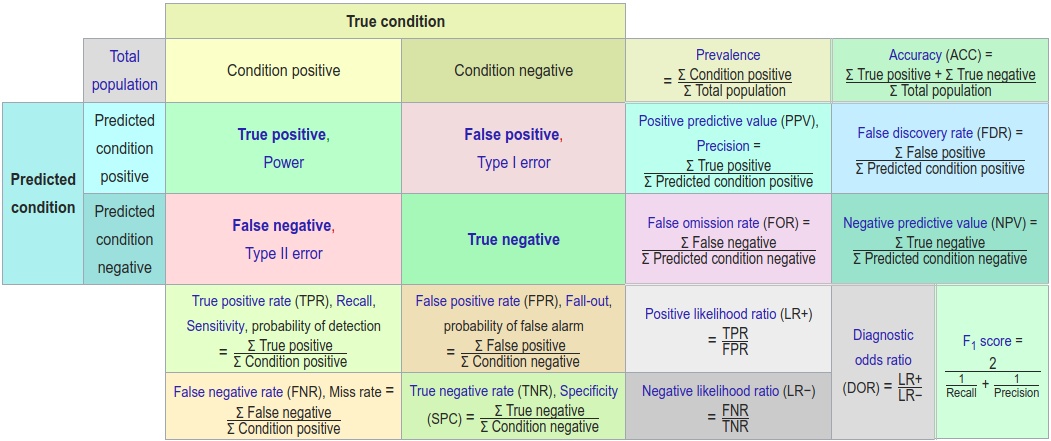R 语言中，调用 caret 包中的 confusionMatrix() 函数可以直接计算上图中的各个统计量。举个两类别例子（取自confusionMatrix()的帮助文档），

lvs <- c("normal", "abnormal")
truth <- factor(rep(lvs, times = c(86, 258)),
levels = rev(lvs))
pred <- factor(
c(
rep(lvs, times = c(54, 32)),
rep(lvs, times = c(27, 231))),
levels = rev(lvs))
xtab <- table(pred, truth)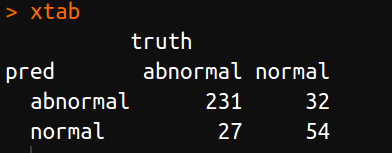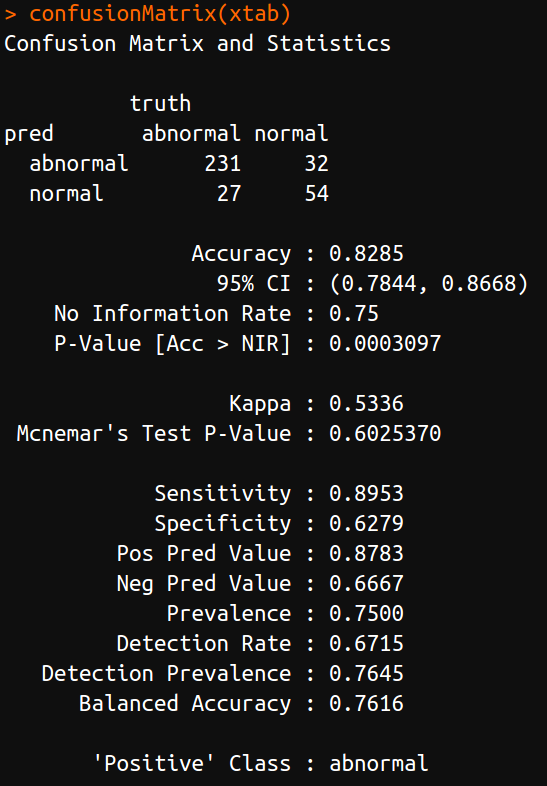ROC 曲线¶

pROC¶

AppliedPredictiveModeling源码包给出的做法是调用 pROC，先计算得到 roc 对象，然后利用其 plot 函数便可以绘制 ROC曲线

library(pROC)
rpartRoc <- roc(response = rpartFit$pred$obs,
predictor = rpartFit$pred$successful,
levels = rev(levels(rpartFit$pred$obs)))
plot(rpartRoc, type = "s", print.thres = c(.5),
print.thres.pch = 3,
print.thres.pattern = "",
print.thres.cex = 1.2,
col = "red", legacy.axes = TRUE,
print.thres.col = "red")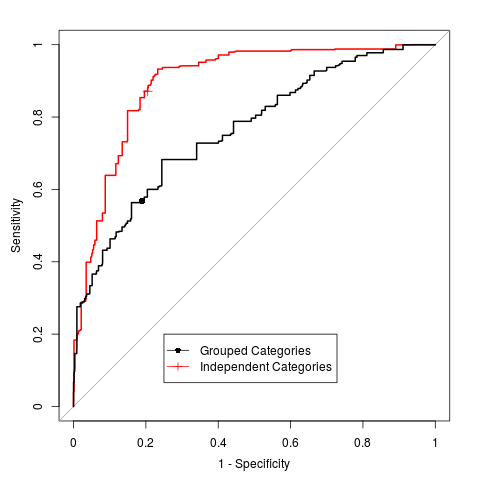ROCR¶

library(ROCR)
rocplot = function(obj, ...) {
predob = prediction(obj$pred$successful,
obj$pred$obs,
label.ordering = rev(levels(obj$pred$obs)))
perf = performance(predob , "tpr" , "fpr")
plot(perf, ...)
}
rocplot(rpartFit, col="red")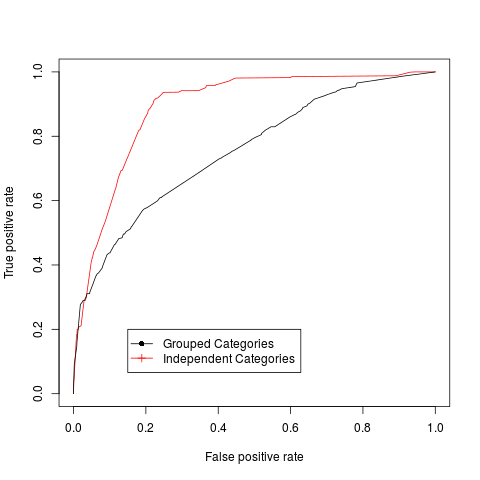比较¶

1. pROC 可以更方便获取相应数据，比如阈值，以及对应 AUC 值，并可以在图象中表示出来。
2. ROCR 绘图横纵坐标的选择更灵活，除了常见的True Positive Rate ~ False Positive Rate，即Sensitivity ~ 1-Specificity 还可以选择 lift ~ rpp